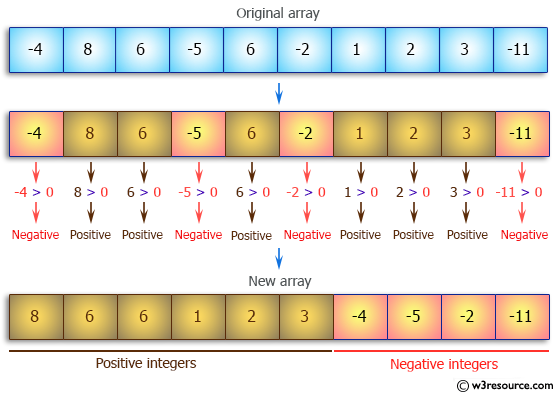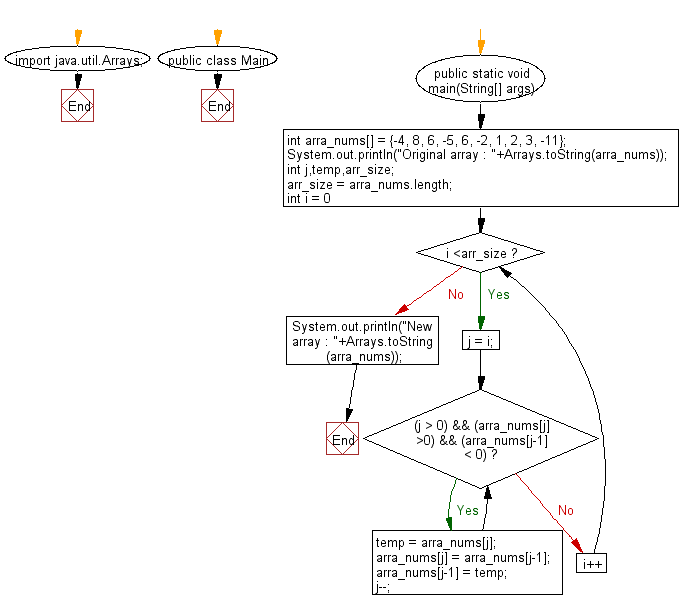﻿ Java: Positive integers come before negative in an array# Java Array Exercises: Arrange the elements of a given array of integers where all positive integers appear before all the negative integers

## Java Array: Exercise-49 with Solution

Write a Java program to arrange the elements of a given array of integers where all positive integers appear before all the negative integers.

Pictorial Presentation:Sample Solution:

Java Code:

``````import java.util.Arrays;
public class Main {

public static void main(String[] args) {
int arra_nums[] = {-4, 8, 6, -5, 6, -2, 1, 2, 3, -11};
System.out.println("Original array : "+Arrays.toString(arra_nums));
int j,temp,arr_size;

arr_size = arra_nums.length;
for (int i = 0; i <arr_size; i++){
j = i;

//Shift positive numbers left, negative numbers right

while ((j > 0) && (arra_nums[j] >0) && (arra_nums[j-1] < 0)){
temp = arra_nums[j];
arra_nums[j] = arra_nums[j-1];
arra_nums[j-1] = temp;
j--;
}
}
System.out.println("New array : "+Arrays.toString(arra_nums));
}
}
```
```

Sample Output:

```Original array : [-4, 8, 6, -5, 6, -2, 1, 2, 3, -11]
New array : [8, 6, 6, 1, 2, 3, -4, -5, -2, -11]
```

Flowchart:Visualize Java code execution (Python Tutor):

Java Code Editor:

Improve this sample solution and post your code through Disqus

What is the difficulty level of this exercise?

Test your Programming skills with w3resource's quiz.

﻿

## Java: Tips of the Day

countOccurrences

Counts the occurrences of a value in an array.

Use Arrays.stream().filter().count() to count total number of values that equals the specified value.

```public static long countOccurrences(int[] numbers, int value) {
return Arrays.stream(numbers)
.filter(number -> number == value)
.count();
}
```

Ref: https://bit.ly/3kCAgLb